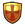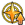1.251 Bài viết

{{Activeskill2page
|img = invishield
|name = Illusion
|rank = A
|servanticons =

|effect =Grants party Invincibility for 1 turn.
Increases party's critical star generation rate for 1 turn.
Reduces all enemies' critical attack chance for 3 turns.
|leveleffect =Star Rate +
|l1 = 30%
|l2 = 32%
|l3 = 34%
|l4 = 36%
|l5 = 38%
|l6 = 40%
|l7 = 42%
|l8 = 44%
|l9 = 46%
|l10 = 50%
|2leveleffect =Crit Chance -
|2l1 = 10%
|2l2 = 11%
|2l3 = 12%
|2l4 = 13%
|2l5 = 14%
|2l6 = 15%
|2l7 = 16%
|2l8 = 17%
|2l9 = 18%
|2l10 = 20%
|c1 = 9
|c2 = 9
|c3 = 9
|c4 = 9
|c5 = 9
|c6 = 8
|c7 = 8
|c8 = 8
|c9 = 8
|c10 = 7
}}

Community content is available under CC-BY-SA unless otherwise noted.